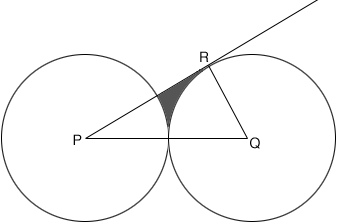SEARCH HOMEMath Central Quandaries & QueriesQuestion from xhesika(jessica), a student: hi!this is the first time for me using this site. I need help with this question Two circles of radius 10 are tangent to each other. A tangent is drawn from the centre of one of the circles to the second circle.To the nearest integer find the area of the shaded region.Hi,

You didn't send a diagram but I think that the shaded region in my diagram is the region in question.If this is the correct diagram I can give you a hint to get started.

What is the measure of the angle QRP?

Write back if you need more assistance,
PennyMath Central is supported by the University of Regina and The Pacific Institute for the Mathematical Sciences.